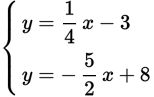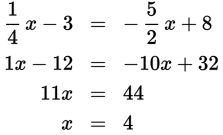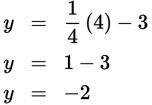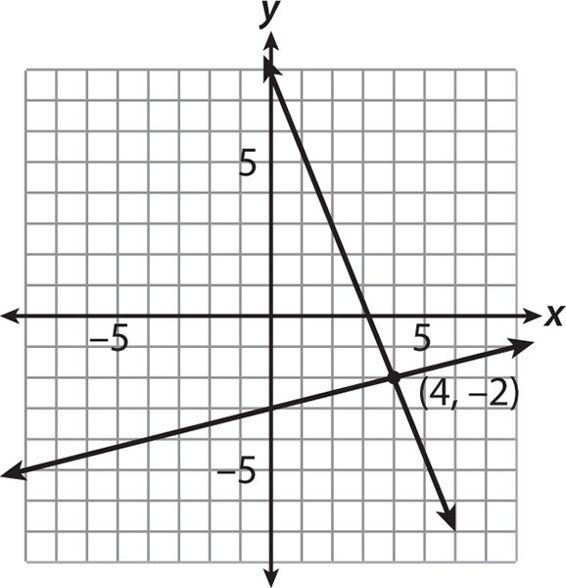# SAT Math Multiple Choice Question 459: Answer and Explanation

### Test Information

Question: 459

9.Which of the following is the y-coordinate of the solution to the system of equations given above?

• A. –8
• B. –2
• C. 2
• D. 4

Explanation:

B

Difficulty: Medium

Category: Heart of Algebra / Systems of Linear Equations

Strategic Advice: This system is set up perfectly to solve using substitution because both equations are already solved for y.

Getting to the Answer: Set both x expressions equal to one another and solve. Multiply the whole equation by 4 first, to get rid of the fractions.The question asks for the y-coordinate of the solution, so substitute 4 for x in either equation and solve for y.As an alternate method, because the equations are already in slope-intercept form, you could graph both equations in your calculator and find the point of intersection, which is (4, –2).